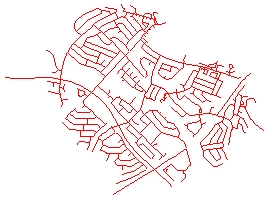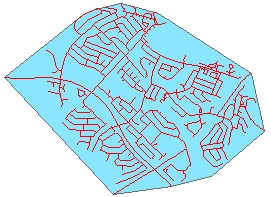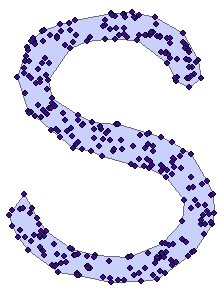## Build Convex Hull

Running programmatically

Builds the Convex Hull of the features of a layer

Convex hull is a polygonal area that is of smallest length and so that any pair of points within the area have the line segment between them contained entirely inside the area.

ConvexNon Convex (Concave)Defining the convex Hull of a set of points is useful, for example in the case of enclosing the points, using a fence of shortest total length.

Source DataConvex HullWhile in general the Convex Hull is good to describe the shape of the input data points, in many cases a polygon that describes better the region occupied by the point cloud is needed. See the Create Concave Hull function

Convex HullConcave Hull### Inputs:

• A feature layer (Point, Multipoint, Polyline, Polygon)

### Outputs:

• New polygon layer.

### Running Programmatically

(Go to TOP)

Parameters

Expression Explanation
Function Name ConvexHull
<input dataset> A String representing the input layer.
<output dataset> A String - the full name of the output layer.

### Running the function

ETGWPath used in the table below is the  full path to ETGWRun.exe (E.G. "C:\Program Files\ETSpatial Techniques\ETGeo Wizards\ETGWRun.exe")

Language Syntax
Python subprocess.call([ETGWPath, "ConvexHull", "input dataset", "output dataset"])
.NET using ETGWRun.exe StartInfo.FileName = ETGWPath
StartInfo.Arguments = "ConvexHull" "input dataset" "output dataset"
.NET using ETGWOutX.dll ConvexHull(input dataset, output dataset)
ArcPy arcpy.ConvexHull(input dataset, output dataset)

Notes:

• <> - required parameter

• {} - optional parameter

• See examples for Python , .NET or ArcPy

• The argument separator for StartInfo.Arguments is space. If a string might contain a space, you need to double quote it.

(Go to TOP)